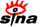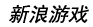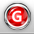下载新浪UT Game　客户端高速下载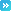推荐：全球新网游 | 网页游戏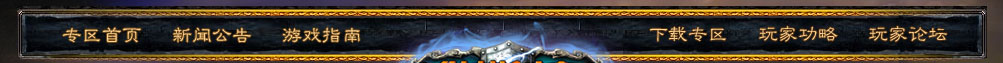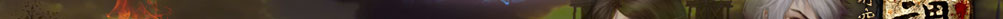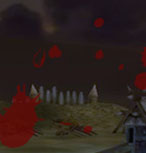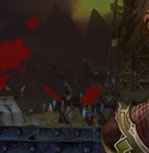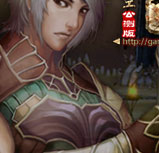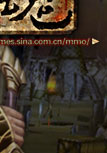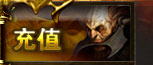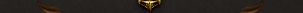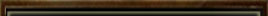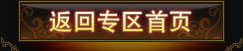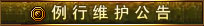为了保证游戏运行的稳定性及提升整体服务质量，《纵横时空》游戏全部服务器将每周二凌晨4：00-12：00进行例行停机维护， 停机期间给您带来的不便，敬请谅解。祝大家游戏愉快！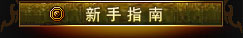登录游戏 创建角色 界面说明 基本操作 快 捷 键 角色属性 系统设置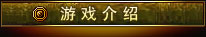背景故事 世界介绍 两大阵营 五大宗派 配置说明 下载安装 收费充值 Vista系统启动指南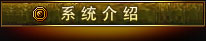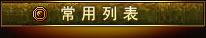游戏问题联系电话：            010-84799696 充值问题联系电话：客服电话：010-52926702/03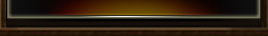# 沙平野

 任务名称 接受等级 给予任务NPC 阵营需求 任务类型 避难营地 >=33 沙无惧 主线任务 蝎人灾祸 >=33 曲光 主线任务 采集药材 >=33 曲光 主线任务 罗汉秘文 >=33 朱湖荒 主线任务 朱湖荒的建议 >=33 朱湖荒 主线任务 勇士的资质 >=33 石原 主线任务 兽人的布署 >=33 石原 主线任务 援军I >=33 石原 新洛 主线任务 援军II >=33 貂云 新洛 主线任务 援军I >=33 石原 正仪 主线任务 援军II >=33 夏辉 正仪 主线任务 引见 >=33 夜全 主线任务 清除苦力 >=33 黄坡 主线任务 蒙面恶匪 >=33 黄坡 主线任务 黄坡的忠告 >=33 黄坡 主线任务 走向迷途之地 >=33 黄坡 主线任务 小希的手艺I >=33 小希 支线任务 小希的手艺II >=33 小希 支线任务 罗汉印记 >=33 夜全 支线任务 蝎人之灾I >=34 马沙 支线任务 蝎人之灾II >=33 朱湖荒 支线任务 蝎人之灾III >=33 朱湖荒 支线任务 石原的秘术I >=35 石原 支线任务 石原的秘术II >=33 石原 支线任务 兽人精锐 >=33 石原 支线任务 清除石兽 >=33 夜全 支线任务 夜全的阵法I >=33 夜全 支线任务 夜全的阵法II >=33 夜全 支线任务 夜全的阵法III >=33 石原 支线任务 醉言醉语 >=33 醉鬼黄三 支线任务 蚁毒之解I >=33 黄坡 支线任务 蚁毒之解II >=33 财第一 支线任务 蚁毒之解III >=33 财第一 支线任务 恶火的财宝I >=33 黄坡 支线任务 恶火的财宝II >=33 古岩明 支线任务 清除巨眼蝎 >=33 曲光 支线任务 锋刀 >=33 朱湖荒 支线任务 斩首行动 >=33 石原 支线任务 石人之王 >=33 夜全 支线任务 斩杀匪首 >=33 财第一 支线任务 石人现象I >=33 石原 支线任务 石人现象II >=33 夜全 支线任务 石人现象III >=33 夜全 支线任务 兑换黄沙徽记 >=0 图伽 支线任务 兑换黄沙徽记 >=0 金景 支线任务 兑换黄沙徽记 >=0 风霆 支线任务 兑换黄沙徽记 >=0 于灰烟 支线任务 兑换黄沙徽记 >=0 赵猛 支线任务 紧急救援 >=35 吕悍 PVP阵营任务 神奇的发现 >=35 新洛 PVP阵营任务 调查开始 >=35 貂云 新洛 PVP阵营任务 历史的痕迹I >=35 徐威 新洛 PVP阵营任务 争分夺秒 >=35 徐威 新洛 PVP阵营任务 历史的痕迹II >=35 韩玉 新洛 PVP阵营任务 自然之力 >=35 韩玉 新洛 PVP阵营任务 神奇的发现 >=35 正仪 PVP阵营任务 调查开始 >=35 夏辉 正仪 PVP阵营任务 历史的痕迹I >=35 杨晁 正仪 PVP阵营任务 争分夺秒 >=35 杨晁 正仪 PVP阵营任务 历史的痕迹II >=35 王征 正仪 PVP阵营任务 自然之力 >=35 王征 正仪 PVP阵营任务 紧急事件 >=33 夏辉 正仪 阵营任务 与夏辉汇合 >=33 洛青剑 正仪 阵营任务 清除石怪 >=33 夏辉 正仪 阵营任务 不自量力 >=33 夏辉 正仪 阵营任务 机密文件 >=33 曲静然 正仪 阵营任务 石人暴动 >=33 夏辉 正仪 阵营任务 石料 >=33 夏辉 正仪 阵营任务 采集药材 >=33 夏辉 正仪 阵营任务 突发事件 >=33 貂云 新洛 阵营任务 汇合 >=33 古战阳 新洛 阵营任务 可恶的石怪 >=33 貂云 新洛 阵营任务 大显身手 >=33 貂云 新洛 阵营任务 秘密文件 >=33 应云 新洛 阵营任务 石人的骚扰 >=33 貂云 新洛 阵营任务 夺回粮食 >=33 貂云 新洛 阵营任务 寻找止血药 >=33 貂云 新洛 阵营任务 带口信 >=33 马沙 支线任务 击退蝎人 >=33 袁一铭 支线任务 求助马沙 >=33 袁一铭 支线任务 马沙的计策 >=33 马沙 支线任务 兽人的末日 >=33 马沙 支线任务 安抚袁一铭 >=33 马沙 支线任务 取药 >=33 袁一铭 支线任务 危机四伏 >=33 袁一铭 支线任务 为村民报仇 >=33 袁一铭 支线任务 回忆往事 >=33 袁一铭 支线任务 叛逆者 >=33 夜全 支线任务 祭拜祖先 >=33 夜全 支线任务 石碑的秘密I >=33 夜全 正仪 支线任务 石碑的秘密II >=33 岩妹 正仪 支线任务 石碑的秘密III >=33 岩妹 正仪 支线任务 石碑的秘密I >=33 夜全 新洛 支线任务 石碑的秘密II >=33 铁悍 新洛 支线任务 石碑的秘密III >=33 铁悍 新洛 支线任务 恼人的石人 >=33 夜全 支线任务 营地的求助 >=33 石原 支线任务 造成混乱 >=33 展刀 支线任务 夺回补给品 >=33 展刀 支线任务 合作I >=35 夏辉 正仪 支线任务 合作II >=35 夜全 正仪 支线任务 合作I >=35 貂云 新洛 支线任务 合作II >=35 夜全 新洛 支线任务 收集草药 >=33 财第一 每日任务 石人暴动 >=33 夜全 每日任务 讨伐兽人 >=35 古岩明 每日任务 收集火蚁卵 >=35 财第一 每日任务 修补房屋 >=35 曲光 每日任务 兽人的骚扰 >=35 沙无惧 每日任务 解毒 >=35 无水 每日任务 匪患 >=35 古岩明 每日任务 谈判归来 >=35 夜全 正仪 每日任务 谈判归来 >=35 夜全 新洛 每日任务 紧急救援 >=35 吕悍 每日任务 军情汇报 >=33+<=36 貂云 新洛 每日任务 罗汉密信 >=35+<=38 夜全 新洛 每日任务 外交活动 >=36+<=38 古岩明 新洛 每日任务 军情汇报 >=33+<=36 夏辉 正仪 每日任务 罗汉密信 >=35+<=38 夜全 正仪 每日任务 外交活动 >=36+<=38 古岩明 正仪 每日任务 不义之财 >=33+<=36 财第一 每日任务 破坏行动 >=35+<=38 朱湖荒 每日任务 消除困扰 >=33+<=39 夏辉 正仪 每日任务 消除叛逆 >=33+<=39 貂云 新洛 每日任务 稀有材料 >=33 财第一 每日任务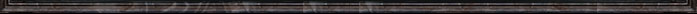留言 更多>>
 本新闻留言共条 显示 条
 登录名： 　密码： 　 匿名发表